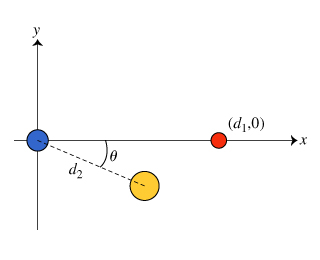# Problem: Consider the following configuration of fixed, uniformly charged spheres (see the figure ):a blue sphere fixed at the origin with positive charge q,a red sphere fixed at the point (d1, 0) with unknown charge qred, anda yellow sphere fixed at the point (d2cos(θ), -d2sin(θ)) with unknown charge qyellow.a) What is the sign on the charge of the yellow sphere? Positive or Negativeb) What is the sign of the charge on the red sphere? Positive or Negativec) What is the sign of the charge on the blue sphere? Positive or Negative

###### FREE Expert Solution

a)

From the information given, the x-component of the position vector of the yellow sphere is positive: d2cosθ

The y- component of the position of the yellow sphere is negative: - d2sinθ

The net electric force on the blue sphere is F = (0, - F), where F > 0

The blue and the red spheres placed along the x-axis have no component in the y-axis.

Therefore, the net forces exerted on the blue sphere is directed along the negative y-axis.

The y component of the yellow sphere's position vector is negative.

97% (173 ratings)###### Problem Details

Consider the following configuration of fixed, uniformly charged spheres (see the figure ):

• a blue sphere fixed at the origin with positive charge q,
• a red sphere fixed at the point (d1, 0) with unknown charge qred, and
• a yellow sphere fixed at the point (d2cos(θ), -d2sin(θ)) with unknown charge qyellow.a) What is the sign on the charge of the yellow sphere? Positive or Negative

b) What is the sign of the charge on the red sphere? Positive or Negative

c) What is the sign of the charge on the blue sphere? Positive or Negative